# Fourier Series Notes | Study Basic Physics for IIT JAM - Physics

## Physics: Fourier Series Notes | Study Basic Physics for IIT JAM - Physics

The document Fourier Series Notes | Study Basic Physics for IIT JAM - Physics is a part of the Physics Course Basic Physics for IIT JAM.
All you need of Physics at this link: Physics

Consider a periodic function f(x) with a period L and let us calculate the Fourier transform of it. We define a new function f0(x)=f(x) in the [0, L] interval and zero otherwise. Then: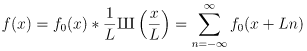Apply Fourier transform: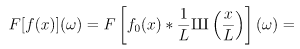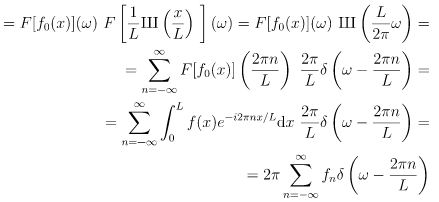where fn are called Fourier coefficients: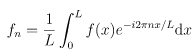We can see that the Fourier transform is zero for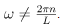. For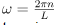it is equal to a delta function times a 2π multiple of a Fourier series coefficient. The delta functions structure is given by the period L of the function f(x). All the information that is stored in the answer is inside the fn coefficients, so those are the only ones that we need to calculate and store.
The function f(x) is calculated from the fn coefficients by applying the inverse Fourier transform to the final result of as follows: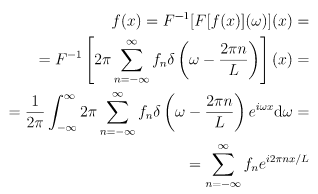The expansion is called a Fourier series. It is given by the Fourier coefficients fn. The equation provides the relation between a Fourier transform and a Fourier series.
For example for f(x) = sin(x), the only nonzero Fourier coefficients for L=2π are f-1 = i/2 and f1 =-i/2  . The Fourier transform then is: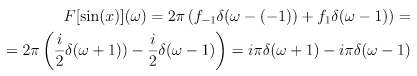For f(x) = 1 the only nonzero Fourier coefficient is f0=1, the Fourier transform then is: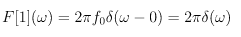For f(x) = e3ix the only nonzero Fourier coefficient for L=2π is f3= 1, the Fourier transform then is: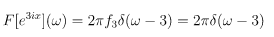For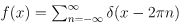the Fourier coefficients for L=2\pi are all equal to fn = 1/2π and the Fourier transform is: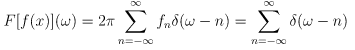Note: if we start from, for simplicity on an interval [-π,π]: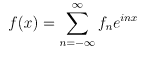To calculate the Fourier coefficients fn, we can just multiply both sides of by e-imx and integrate: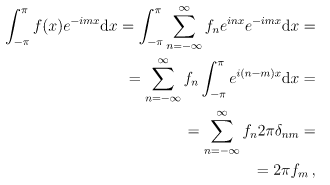so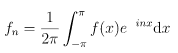The document Fourier Series Notes | Study Basic Physics for IIT JAM - Physics is a part of the Physics Course Basic Physics for IIT JAM.
All you need of Physics at this link: PhysicsUse Code STAYHOME200 and get INR 200 additional OFF

## Basic Physics for IIT JAM

220 videos|156 docs|94 tests

Track your progress, build streaks, highlight & save important lessons and more!

,

,

,

,

,

,

,

,

,

,

,

,

,

,

,

,

,

,

,

,

,

;